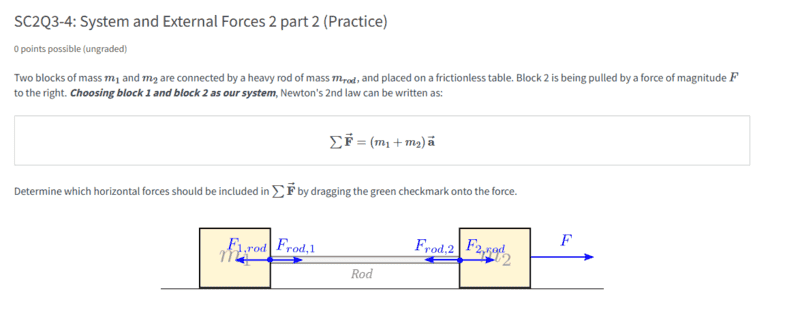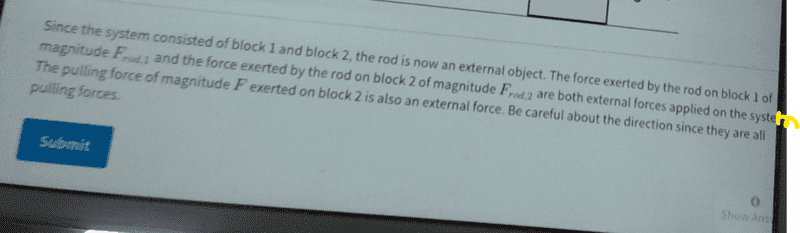# Doubt about solution -- Two masses connected by a rod moving on a surface

• String theory guy
String theory guy
Homework Statement
The statement is below.
Relevant Equations
Newton's Second LawThey say that all the forces are pulling forces? How is this the case?

How would this setup even work? I don't think block 2 could pull the rod.

Homework Helper
Gold Member
2022 Award
They say that all the forces are pulling forces? How is this the case?
Can you find one that isn't?

•String theory guy
String theory guy
Yes I can @haruspex :
F1, rod
F2, rod

Homework Helper
Gold Member
2022 Award
Yes I can @haruspex :
F1, rod
F2, rod
F1,rod is the force m1 exerts on the rod. That force pulls the rod to the left.

•String theory guy
String theory guy
F1,rod is the force m1 exerts on the rod. That force pulls the rod to the left.
I thought it was the opposite way round. How did you derive that @haruspex ?

Homework Helper
Gold Member
2022 Award
I thought it was the opposite way round. How did you derive that @haruspex ?
Do you mean, how do I know that F1,rod is the force m1 exerts on the rod, not the force the rod exerts on m1? Because clearly everything does move to the right, and the only way m1 is going to move to the right is if the force the rod exerts on m1 is to the right. So that must be Frod,1.
But the question ought to have made the notation clear.

•String theory guy
String theory guy
Do you mean, how do I know that F1,rod is the force m1 exerts on the rod, not the force the rod exerts on m1? Because clearly everything does move to the right, and the only way m1 is going to move to the right is if the force the rod exerts on m1 is to the right. So that must be Frod,1.
But the question ought to have made the notation clear.
Yea that is my doubt I think @haruspex - how dose everything in the system move to the right? Is there some glue or something between the block and masses? That is the only way I can see mass 1 moving to the right being in contact with the rod that is moving to the right.

I don't know, but the force of static friction should not be that great allowing the rod to pull mass 1.

•